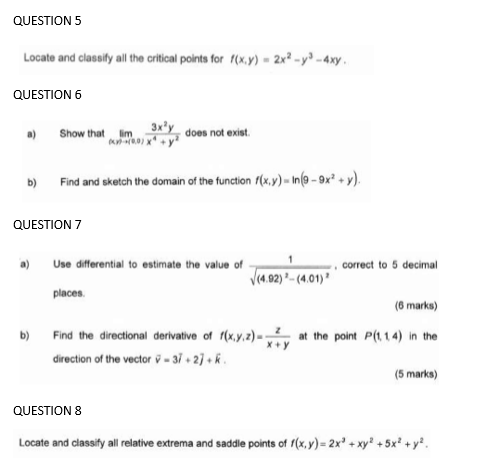### Create an Account

Already have account?

### Forgot Your Password ?

Home / Questions / QUESTION 5 Locate and classify all the critical points for "(x,y) - 2x - y) - 4xy QUESTION...

# QUESTION 5 Locate and classify all the critical points for "(x,y) - 2x - y) - 4xy QUESTION 6 Show that tim 0.01 3x®y, does not exist b) Find and sketch the domain of the function f(x,y) - In (9 - 9x +

QUESTION 5 Locate and classify all the critical points for "(x,y) - 2x - y) - 4xy QUESTION 6 Show that tim 0.01 3x®y, does not exist b) Find and sketch the domain of the function f(x,y) - In (9 - 9x + y). QUESTION 7 a) Use differential to estimate the value of correct to 5 decimal (4,92) - (4:01) places (6 marks) Find the directional derivative of f(x,y,z) ? at the point P(114) In the direction of the vector v - 37. 27+ (5 marks) b) QUESTION 8 Locate and classify all relative extrema and saddle points of f(x,y)=2x + xy + 5x +y?Apr 14 2021 View more View Less

#### Answer (Solved)Subscribe To Get Solution